Question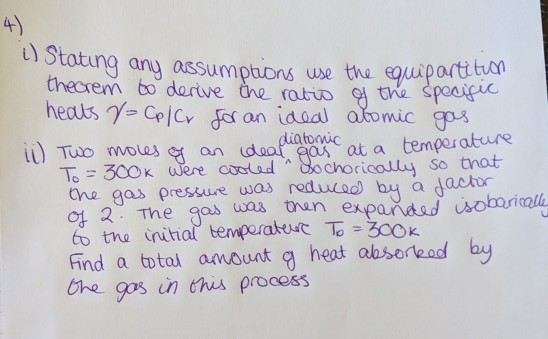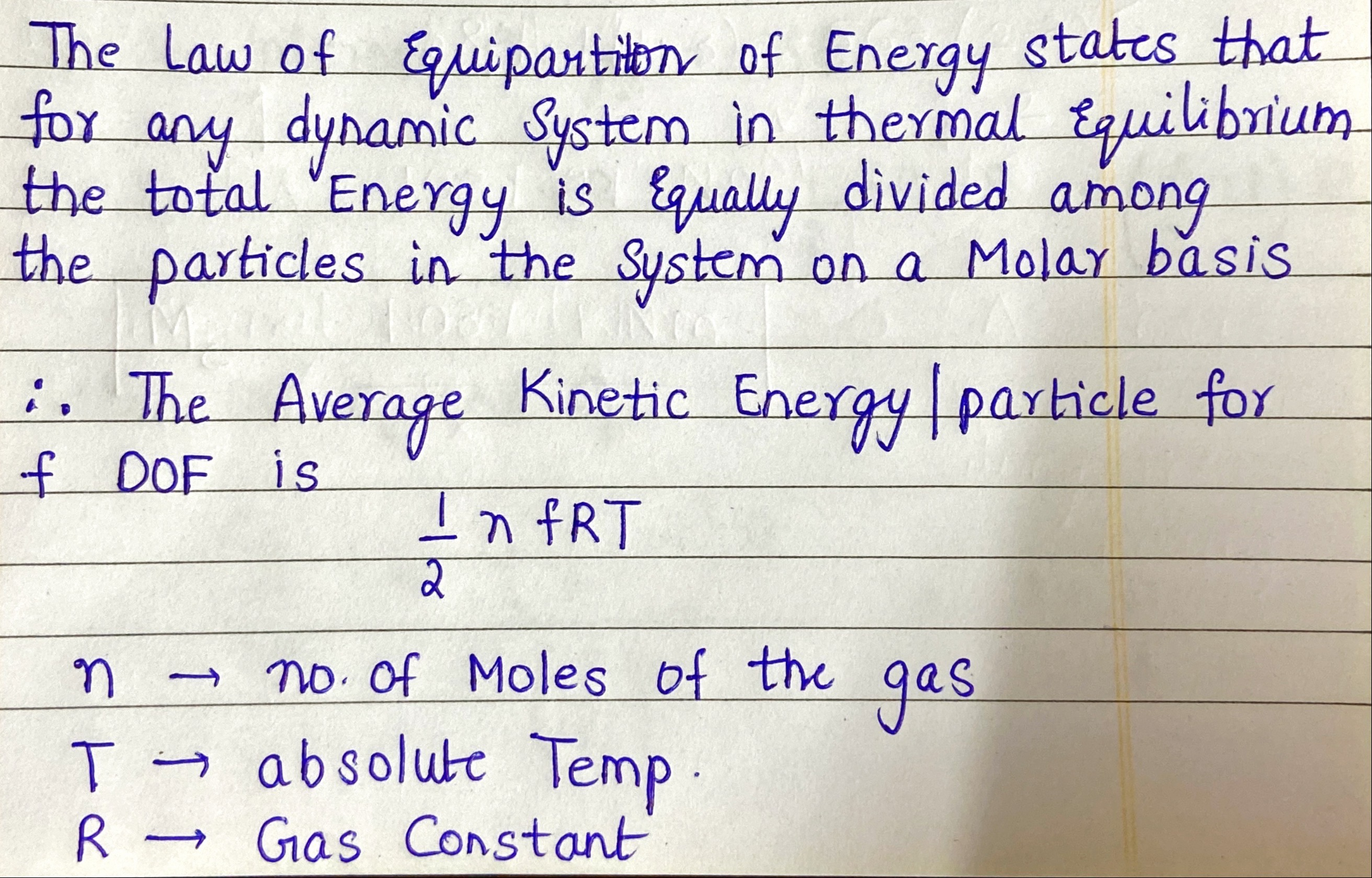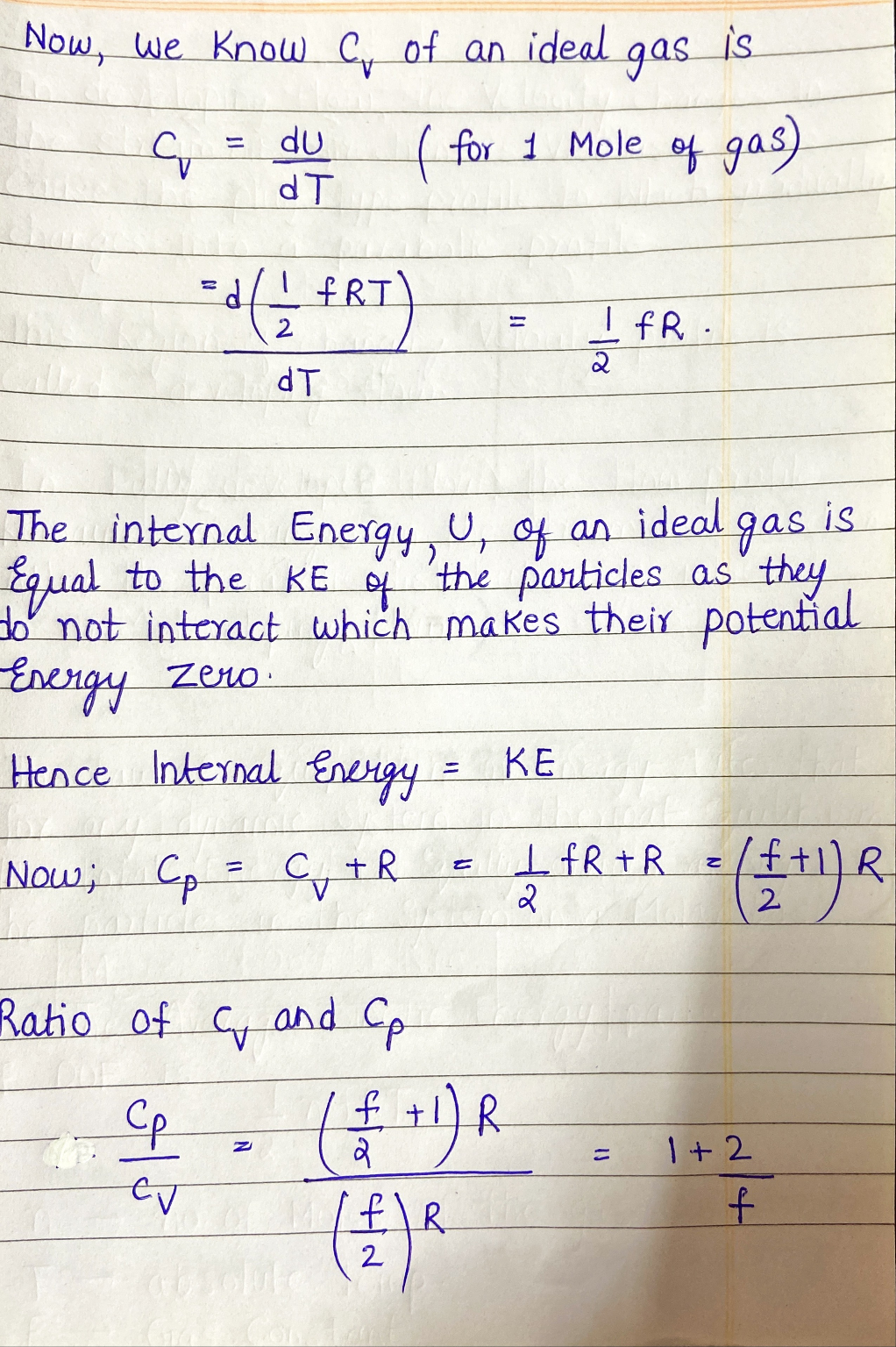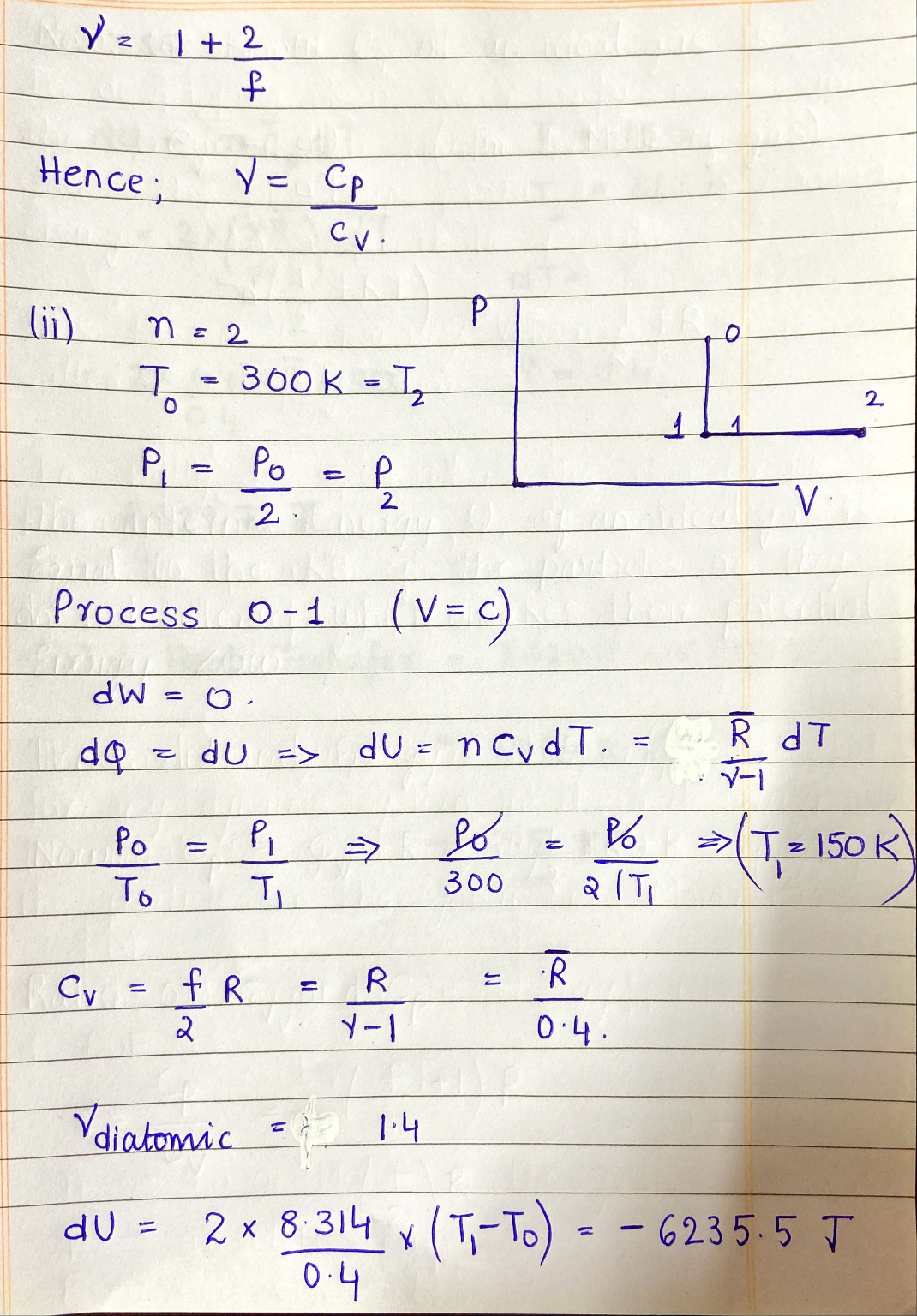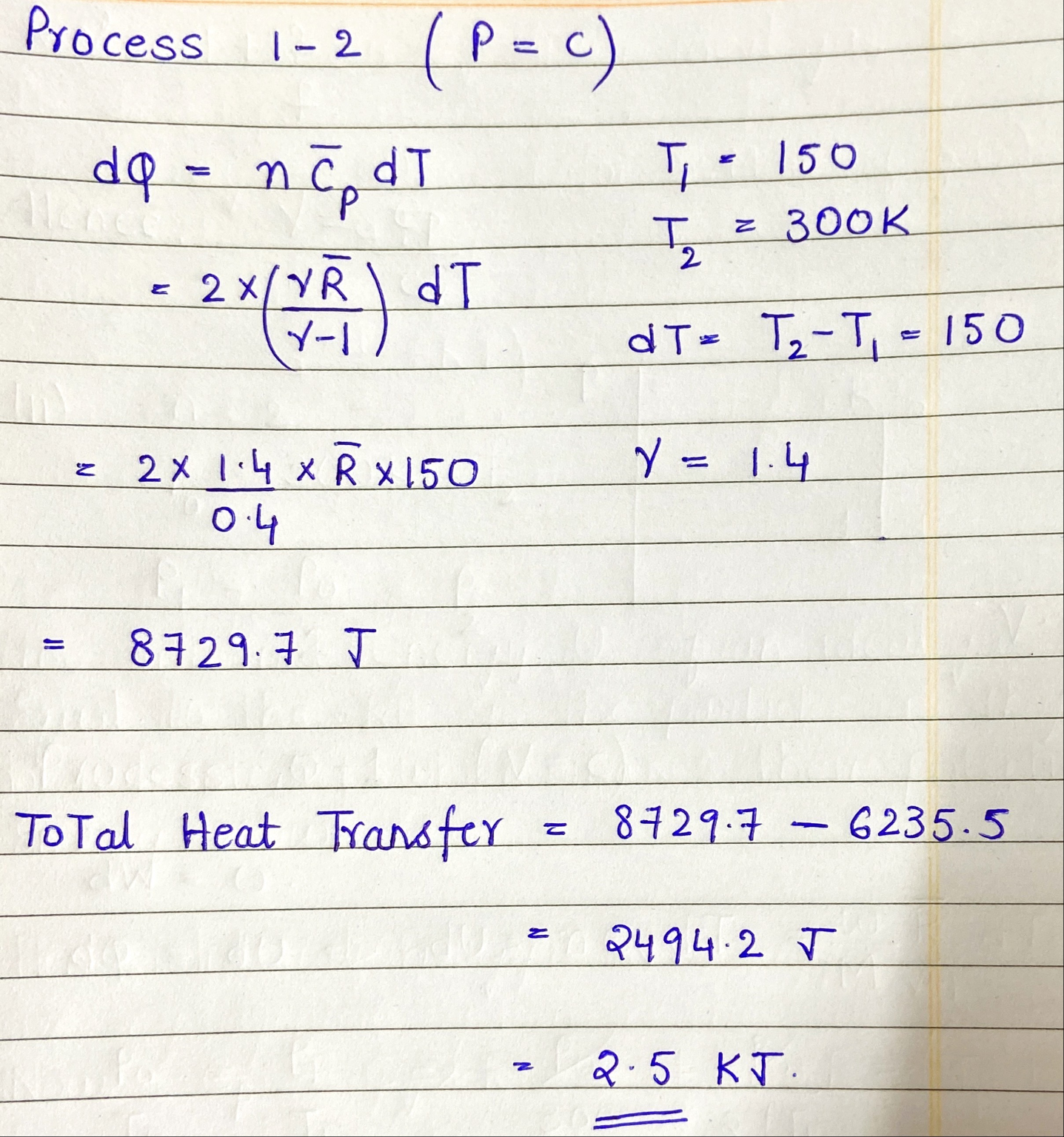#### Earn Coins

Coins can be redeemed for fabulous gifts.

Similar Homework Help Questions
• ### Required information An ideal Otto cycle has a compression ratio of 8. At the beginning of...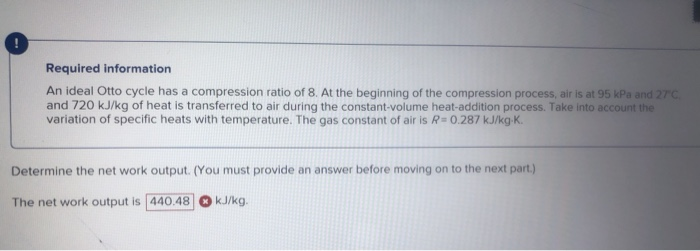Required information An ideal Otto cycle has a compression ratio of 8. At the beginning of the compression process, air is at 95 kPa and 27 and 720 kJ/kg of heat is transferred to air during the constant volume heat addition process. Take into account the variation of specific heats with temperature. The gas constant of air is R=0.287 kJ/kg.K. Determine the network output (You must provide an answer before moving on to the next part.) The net work output...

• ### 4. An ideal gas with constant specific heats undergoes a process from an initial pressure of...

4. An ideal gas with constant specific heats undergoes a process from an initial pressure of 50 kPa and initial specific volume of 4 m^3/kg to a final pressure of 80 kPa and final specific volume of 5 m^3/kg. The mass of the carbon monoxide is 3 kg. The gas has a molar mass of 44 kg/kmol and a specific heat at constant volume of 0.98 kJ/(kg∙K). Determine the entropy change of the gas during the process in kJ/K.

• ### Do problem 9.128E using variable specific heat assumptions instead of constant. Please don't use constant specific...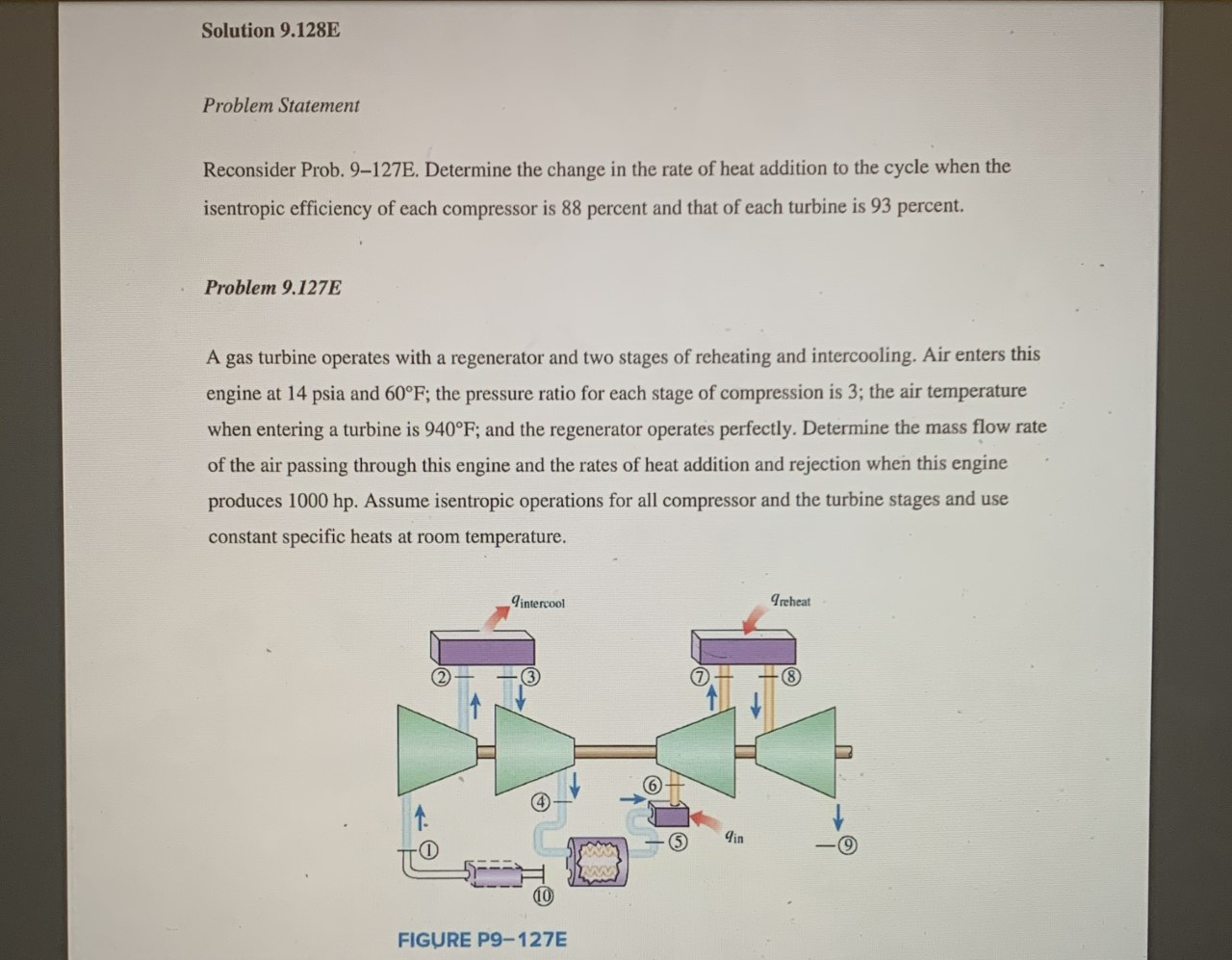Do problem 9.128E using variable specific heat assumptions instead of constant. Please don't use constant specific heat assumption Solution 9.128E Problem Statement Reconsider Prob. 9–127E. Determine the change in the rate of heat addition to the cycle when the isentropic efficiency of each compressor is 88 percent and that of each turbine is 93 percent. Problem 9.127E A gas turbine operates with a regenerator and two stages of reheating and intercooling. Air enters this engine at 14 psia and 60°F;...

• ### 1) Calculate  the  heat  through  a  glass  window  that  is 30.0  cm*150  cm  in  area  and  1.20  mm&n

1) Calculate  the  heat  through  a  glass  window  that  is 30.0  cm*150  cm  in  area  and  1.20  mm  thick.  Assume  the temperature onthe inside of the window is 25.0�C while the outside temperature is 8.00�C. 2)Five hundred joules of heat is absorbed by a system that does 200 J of work on its surroundings. What is the change in the internal energy of the system?    3)An ideal gas expands isothermally, performing 8.80 kJ of work  in  the  process.  Calculate  the  heat  absorbed  during  the expansion. 4)A gas contained in a cylinder that has a piston is kept at a  constant  pressure  of  2.80*10^5Pa. The  gas  expands  from0.500 m^3to 1.50 m^3 when 300 kJ...

• ### Show all your work, thanks! 18. A sample of helium gas (mass of 2.658x10-3kg), is expanded...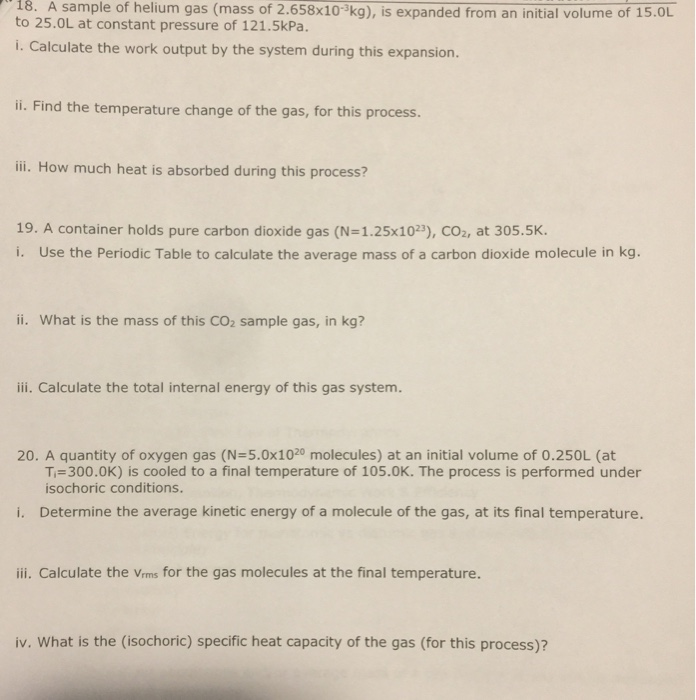Show all your work, thanks! 18. A sample of helium gas (mass of 2.658x10-3kg), is expanded from an initial volume of 15.OL to 25.OL at constant pressure of 121.5kPa. i. Calculate the work output by the system during this expansion. i. Find the temperature change of the gas, for this process. ili. How much heat is absorbed during this process? 19. A container holds pure carbon dioxide gas (N=1.25x10», СОг, at 305.5K. i. Use the Periodic Table to calculate the...

• ### Physical Chemistry Homework

15.1. 2nd Law 1(Points: 5)Once equilibrium is reached, the entropy reaches its maximum value and stays at that value.TrueFalseSave Answer2. 2nd Law 2(Points: 5)The state of equilibrium is also the likeliest one.TrueFalseSave Answer3. Entropy vs. number of states(Points: 10)Suppose the entropy of a protein residue is kB ln(2). What is the number of states available to that residue? (Note that the number of states does not have to beinteger. )1.Save Answer4. Boltzmann Distribution(Points: 10)Suppose the ratio of nitrogen (N2) to...

• ### A gasoline engine operates on the air standard Otto cycle. The air intake to the engine...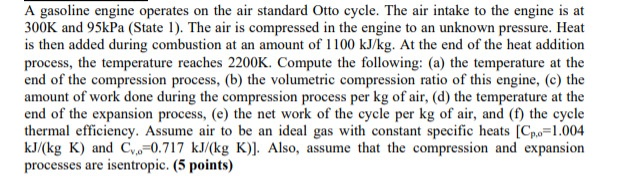A gasoline engine operates on the air standard Otto cycle. The air intake to the engine is at 300K and 95kPa (State 1). The air is compressed in the engine to an unknown pressure. Heat is then added during combustion at an amount of 1100 kJ/kg. At the end of the heat addition process, the temperature reaches 2200K. Compute the following: (a) the temperature at the end of the compression process, (b) the volumetric compression ratio of this engine, (c)...

• ### 4-/6.25 points My Notes SerCP10 12.P.023. An ideal monatomic gas is contained in a vessel of constant volume 0.260 m3....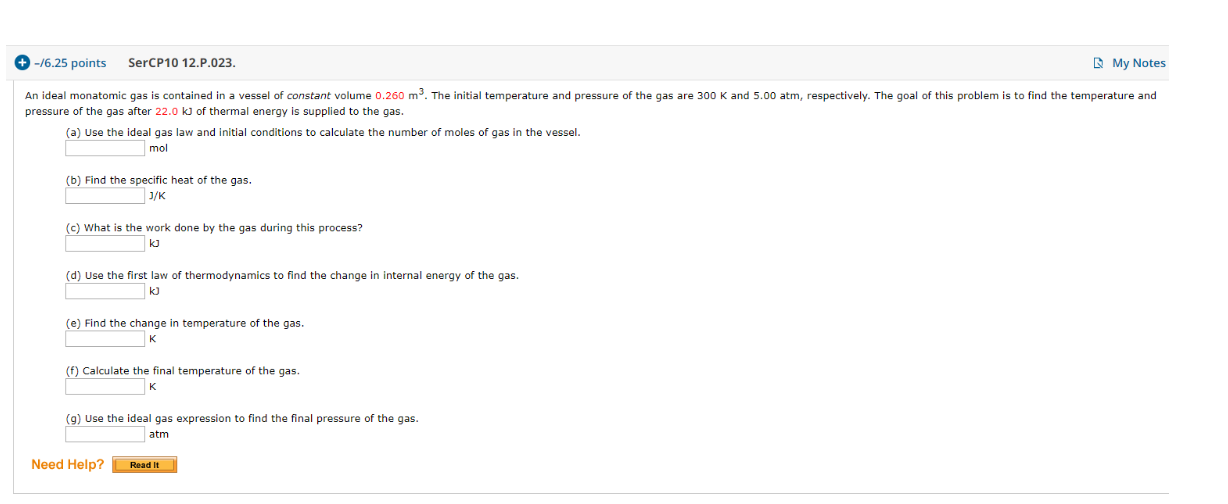4-/6.25 points My Notes SerCP10 12.P.023. An ideal monatomic gas is contained in a vessel of constant volume 0.260 m3. The initial temperature and pressure of the gas are 300 K and 5.00 atm, respectively. The goal of this problem is to find the temperature and pressure of the gas after 22.0 kJ of thermal energy is supplied to the gas. (a) Use the ideal gas law and initial conditions to calculate the number of moles of gas in the...

• ### 4. An Ideal diesel engine has a compression ratio of 20 and uses air as the...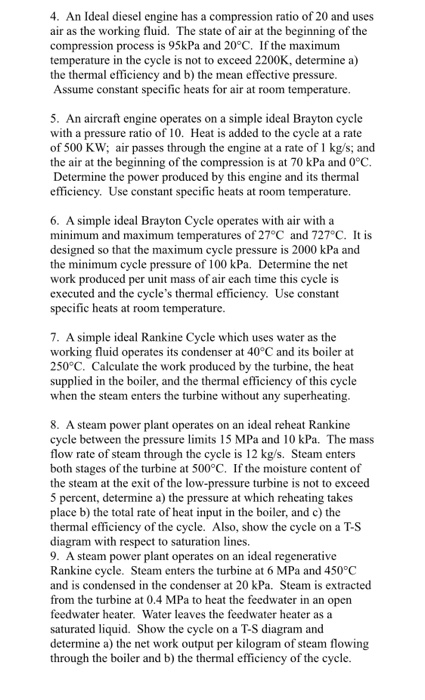4. An Ideal diesel engine has a compression ratio of 20 and uses air as the working fluid. The state of air at the beginning of the compression process is 95kPa and 20°C. If the maximum temperature in the cycle is not to exceed 2200K, determine a) the thermal efficiency and b) the mean effective pressure. Assume constant specific heats for air at room temperature. 5. An aircraft engine operates on a simple ideal Brayton cycle with a pressure ratio...

• ### Problem 4 Consider a gas power cycle executed in a closed system with 0.08 kg of...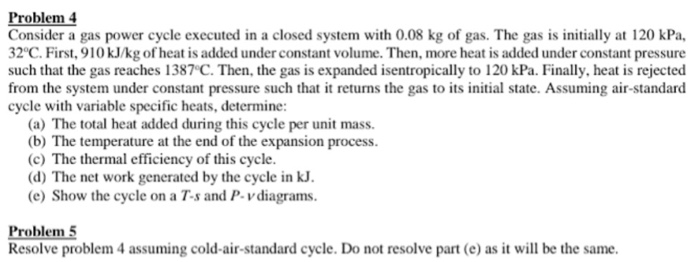Problem 4 Consider a gas power cycle executed in a closed system with 0.08 kg of gas. The gas is initially at 120 kPa, 2°C. First,910 kJ/kg of heat is added under constant volume. Then, more heat is added under constant pressure such that the gas reaches 1387°C. Then, the gas is expanded isentropically to 120 kPa. Finally, heat is rejected from the system under constant pressure such that it returns the gas to its initial state. Assuming air-standard cycle...